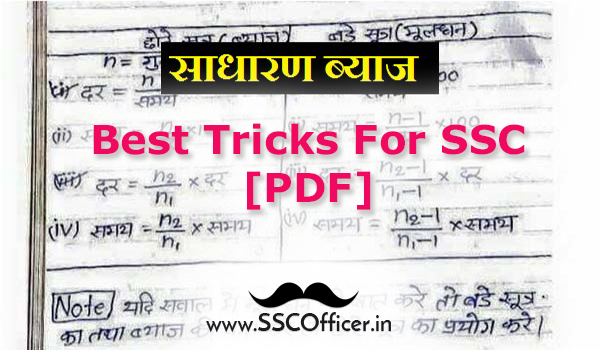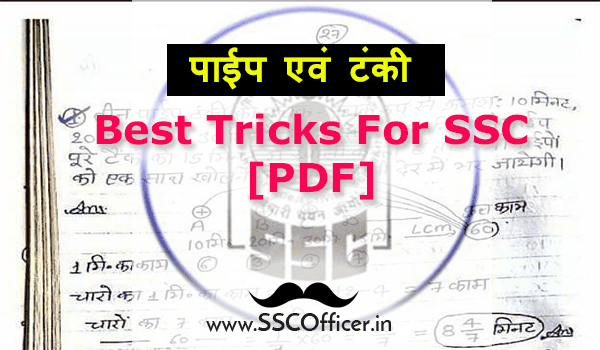## Posts

Showing posts with the label Maths Handwritten Notes

### Advance Maths Special Tricks Handwritten Notes [PDF]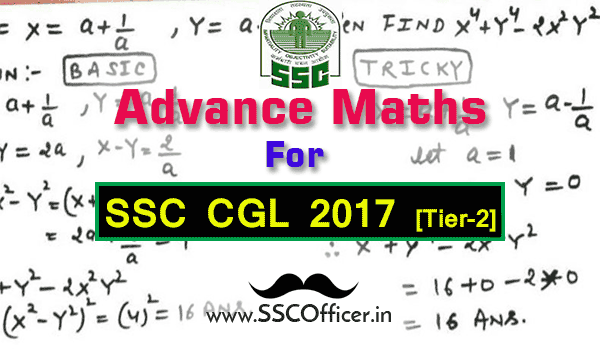Hello Wanna Be Officers, Advance Maths is the important Marks Gainer in SSC CGL Tier-2 Where you required more focus on Maths subject. We have come with an Advance Maths PDF by Expert. You can easily download the PDF and learn easily from this. Download the PDF and Start Learning them. All the Best :) Math PDF By SSC OFFICER Hand Written Math Notes for SSC CGL & CHSL [PDF] Number Series Question Bank  with Solutions [PDF] SSC Maths Handwritten Notes- Free Download [PDF] Data Interpretation (Pie Chart)  Question Bank With Solution [PDF]   All 1300 Advance Math Formulas for SCC Exam [PDF]   Profit and Loss  Handwritten Notes For SSC in Hindi [PDF] Simple Interest Handwritten Notes For SSC [PDF] Pipe & Cistern Best Tricks For SSC Exams [PDF] Kiran's SSC Maths Chapter-wise  Solved Papers [Book-PDF] Mixture & Allegation Shortcut Tricks in Hindi For SSC [PDF] Hand-Written  Algebra Notes  For SSC [PDF] Previous Year

### Average (औसत) Solved Questions in Hindi For SSC Exams [PDF]Hello, Wanna Be Officers, The Average is the important part of Quantitative Aptitude that can boost your marks in SSC Exams. The Average is very important for various exams, it's very easy to understand and quick to learn in less time. Learn this PDF and crack the maths Average section easily in various SSC Exams Download the PDF and Start Learning them. All the Best :) Math PDF By SSC OFFICER Hand Written Math Notes for SSC CGL & CHSL [PDF] Number Series Question Bank  with Solutions [PDF] SSC Maths Handwritten Notes- Free Download [PDF] Data Interpretation (Pie Chart)  Question Bank With Solution [PDF]   All 1300 Advance Math Formulas for SCC Exam [PDF]   Profit and Loss  Handwritten Notes For SSC in Hindi [PDF] Simple Interest Handwritten Notes For SSC [PDF] Pipe & Cistern Best Tricks For SSC Exams [PDF] Kiran's SSC Maths Chapter-wise  Solved Papers [Book-PDF] Mixture & Allegation Shortcut Tricks in Hindi For SSC [P

### Triangle Notes for SSC CGL Exams [PDF]Hello, Wanna Be Officers, The Triangle is the important part of SSC that can boost your marks in SSC Exams. The Triangle is very important for various exams, it's very easy to understand and quick to learn in less time. Learn this PDF and crack the Triangle section easily in various SSC Exams Download the PDF and Start Learning them. All the Best :) Math PDF By SSC OFFICER Hand Written Math Notes for SSC CGL & CHSL [PDF] Number Series Question Bank  with Solutions [PDF] SSC Maths Handwritten Notes- Free Download [PDF] Data Interpretation (Pie Chart)  Question Bank With Solution [PDF]   All 1300 Advance Math Formulas for SCC Exam [PDF]   Profit and Loss  Handwritten Notes For SSC in Hindi [PDF] Simple Interest Handwritten Notes For SSC [PDF] Pipe & Cistern Best Tricks For SSC Exams [PDF] Kiran's SSC Maths Chapter-wise  Solved Papers [Book-PDF] Mixture & Allegation Shortcut Tricks in Hindi For SSC [PDF] Hand-Writte

### Hand-written Notes of Geometry for SSC Exams [PDF]Hello, Wanna Be Officers, Geometry is the important part of mathematics that can boost your marks in SSC Exams. Geometry is very important for various calculation and it's very easy to understand. The problems are easily solved by applying Tricks. We are giving you the Hand Written Notes of Geometry section which is easily understandable and quick to learn in less time. Solve this PDF and crack the maths Geometry section easily in various SSC Exams Download the PDF and Start Learning them. All the Best :) Math PDF By SSC OFFICER Hand Written Math Notes for SSC CGL & CHSL [PDF] Number Series Question Bank  with Solutions [PDF] SSC Maths Handwritten Notes- Free Download [PDF] Data Interpretation (Pie Chart)  Question Bank With Solution [PDF]   All 1300 Advance Math Formulas for SCC Exam [PDF]   Profit and Loss  Handwritten Notes For SSC in Hindi [PDF] Simple Interest Handwritten Notes For SSC [PDF] Pipe & Cistern Best Tricks For SSC

### Question Bank of Number System for SSC Exams [PDF]Hello, Wanna Be Officers, Number System is the important part of mathematics that can boost your marks in SSC Exams. Number System is very important for various calculation and it's very easy to understand. The problems are easily solved by applying Tricks. We are giving you the question bank of Number System section which is easily understandable and quick to learn in less time. Solve this PDF and crack the maths Number System section easily in various SSC Exams Download the PDF and Start Learning them. All the Best :) Math PDF By SSC OFFICER Hand Written Math Notes for SSC CGL & CHSL [PDF] Number Series Question Bank  with Solutions [PDF] SSC Maths Handwritten Notes- Free Download [PDF] Data Interpretation (Pie Chart)  Question Bank With Solution [PDF]   All 1300 Advance Math Formulas for SCC Exam [PDF]   Profit and Loss  Handwritten Notes For SSC in Hindi [PDF] Simple Interest Handwritten Notes For SSC [PDF] Pipe & Cistern Best T

### Hand-Written Algebra Notes For SSC Exams [PDF]Hello, Wanna Be Officers, Algebra is the important part of mathematics that can boost your marks in SSC Exams. Algebra is very important for various calculation and it's very easy to understand. The problems are easily solved by applying Algebra. We are giving you the Hand-written Notes of Algebra section which is easily understandable and quick to learn in less time. Solve this Algebra and crack the maths Algebra section easily in various SSC Exams Download the PDF and Start Learning them. All the Best :) Math PDF By SSC OFFICER Hand Written Math Notes for SSC CGL & CHSL [PDF] Number Series Question Bank  with Solutions [PDF] SSC Maths Handwritten Notes- Free Download [PDF] Data Interpretation (Pie Chart)  Question Bank With Solution [PDF]   All 1300 Advance Math Formulas for SCC Exam [PDF]   Profit and Loss  Handwritten Notes For SSC in Hindi [PDF] Simple Interest Handwritten Notes For SSC [PDF] Pipe & Cistern Best Tricks For SSC E

### Book-PDF: Class Notes of Maths by Rakesh Yadav For SSC Exams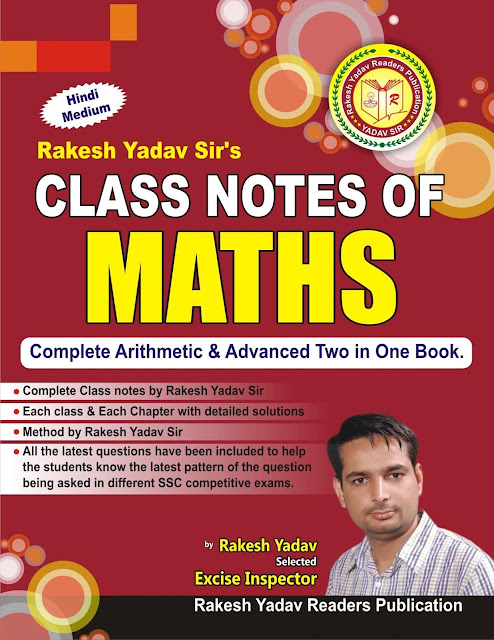Hello Wanna Be Officers, Here we come with a new SSC exams material PDF to get marks easily in exams. Class Notes of Maths by Rakesh Yadav is the important book for SSC Exams and you can easily learn from this book. Now we have posted a PDF of Class Notes of Maths by Rakesh Yadav for SSC Exams. The PDF is free and easy to download by just clicking on below link. The questions are in the chapter-wise arrangement so you individually learn many topics. The previous year papers are included in this book and solved by an expert team so can get good solution and tricks. Download the PDF and Start Learning them. All the Best :) Math PDF By SSC OFFICER Hand Written Math Notes for SSC CGL & CHSL [PDF] Number Series Question Bank  with Solutions [PDF] SSC Maths Handwritten Notes- Free Download [PDF] Data Interpretation (Pie Chart)  Question Bank With Solution [PDF]   All 1300 Advance Math Formulas for SCC Exam [PDF]   Profit and Loss  Handwritten Notes For

### Short-Tricks to solve Clock Questions For SSC Exams [PDF]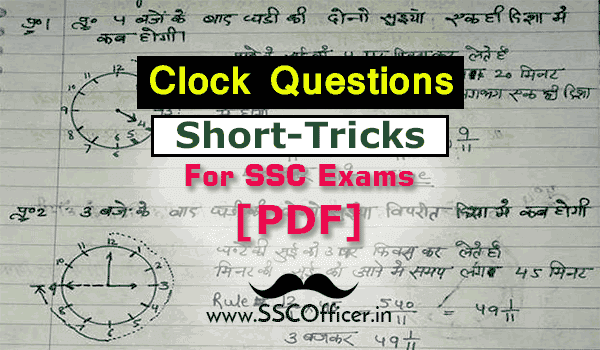Hello Wanna Be Officers, Clock questions are very much important for various SSC Exams from previous two or three years. Basic Concept Of Clock Questions is compiled in a PDF. You can easily download the PDF from below direct link. The tricks are very much important for the SSC CGL and SSC CHSL Exams. First, you have to download the PDF and then start solving the questions one by one to understand the tricks for every type of questions. Download the PDF and Start Learning them. All the Best :) Math PDF By SSC OFFICER Hand Written Math Notes for SSC CGL & CHSL [PDF] Number Series Question Bank  with Solutions [PDF] SSC Maths Handwritten Notes- Free Download [PDF] Data Interpretation (Pie Chart)  Question Bank With Solution [PDF]   All 1300 Advance Math Formulas for SCC Exam [PDF]   Profit and Loss  Handwritten Notes For SSC in Hindi [PDF] Simple Interest Handwritten Notes For SSC [PDF] Pipe & Cistern Best Tricks For SSC Exams [PDF] Kiran

### [PDF] Profit and Loss Handwritten Notes For SSC in Hindi- Free Download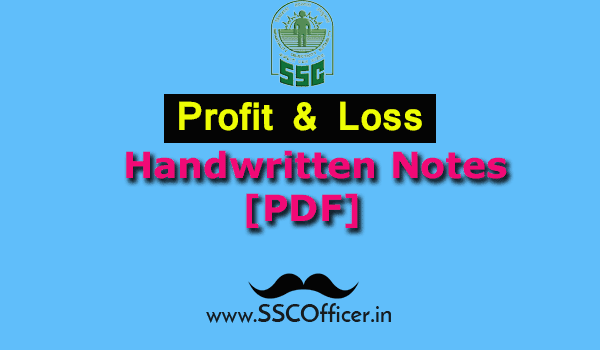Hello Wanna Be Officers, We come with a new SSC Maths Material to score well in Various SSC Exams. This Time we are the focus on the Profit and Loss topic for SSC Exams. In this PDF you can find the Handwritten Notes, Formulas and Tricks to solve Profit and Loss Problem. SSC Officer very much committed to giving you best material. Yon can download the PDF from below link and start learning them. All the Best.:) GK (Hindi) PDF BY SSC OFFICER Hindi- 5000 GK Questions Asked in SSC Exam [PDF] Hindi - 100 General Science GK Questions for SSC [PDF]   Hindi - Geography Handwritten Notes For SSC [PDF]   Hindi Handwritten G.K. Tricks For SSC Exams- [PDF]  GK (English) PDF BY SSC OFFICER 800 GK Questions Asked in IBPS Exams [PDF] Important 800 GK Questions Asked in 2016 Exams [PDF] 5000 GK Questions Asked in SSC Exams [PDF] 5000 GK Questions Asked in SSC Exams - 2 [PDF] Complete Ancient History Notes for SSC CGL [PDF] All in One Must have Capsule for Ban